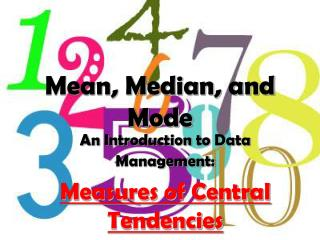DownloadDownload PresentationMean, Median, and Mode

# Mean, Median, and Mode

Download Presentation## Mean, Median, and Mode

- - - - - - - - - - - - - - - - - - - - - - - - - - - E N D - - - - - - - - - - - - - - - - - - - - - - - - - - -
##### Presentation Transcript

1. Mean, Median, and Mode An Introduction to Data Management: Measures of Central Tendencies

2. Why Analyze Data? Data is collected to answer questions. When we want to answer a question, we collect data to provide information on that topic. We take the collected data and analyze it to find out if there are any relationships. DATA IS COLLECTED TO FIND ANSWERS TO MANY DIFFERENT QUESTIONS.

3. The Mean The sum of a list of numbers, divided by the total number of numbers in that list

4. Example • Find the mean of 10, 12, 14, 17, 20. • Sum = 10 + 12 + 14 + 17 + 20 • Sum = 73 • Mean = 73 ÷ 5 • Mean = 14.6

5. Find The MeanSHOW YOUR WORK –Round to 1 decimal place! • {8, 9, 12, 16, 18} • {1, 2, 4, 4, 5, 7, 11} • {25, 26, 27, 36, 42, 52} • {120, 134, 165, 210, 315, 356}

6. Find The Mean • {8, 9, 12, 16, 18} Sum = 8+9+12+16+18 = 63 Mean = 63÷5 = 12.6 • {1, 2, 4, 4, 5, 7, 11} Mean = 34÷5 = 4.9 3. {25, 26, 27, 36, 42, 52} Mean= 209÷6 = 34.8 4. {120, 134, 165, 210, 315, 356} Mean = 1300÷5 = 216.7

7. The Median The middle value in an ordered list of numbers

8. How To Find The Median • Place the numbers in order, from least to greatest. • 0, 1, 2, 3, 4, 4,4 • Find the number that is in the middle of the data set • 0, 1, 2, 3, 4, 4, 4 • 3 is the median of this data set.

9. Example 1 • Find the median of 10, 13, 8, 7, 12. • Order: 7, 8, 10, 12, 13 • Median = 10

10. Oh Oh!!! What do you do if there are an even amount of numbers in your data set? < 2 middle numbers > {10, 12, 16, 18, 20, 24} You take the mean of the two middle values. 16+18 = 34÷2 = 17 The median of this data set is 17.

11. Example 2 • Find the median of 44, 46, 39, 50, 39, 40. • Order: 39, 39, 40, 44, 46, 50 • Median = (40 + 44) ÷ 2 = 42

12. Find The Median • {8, 9, 12, 16, 18} • {4, 2, 6, 4, 1, 7, 11} • {25, 26, 27, 36, 42, 52} • {120, 134, 165, 210, 315, 356}

13. Find The Median • {8, 9, 12, 16, 18} 8, 9, 12, 16, 18 • {4, 2, 6, 4, 1, 7, 11} 1,2, 4,4, 6, 7, 11 3. {25, 26, 27, 36, 42, 52} 25, 26,27,36, 42, 52  (27+36)÷2 =31.5 • {120, 134, 165, 210, 315, 356} 120, 134,165,210, 315, 356  (165 + 210 )÷2 =187.5

14. The Mode The most common value or the value with the highest frequency in a data set.

15. Example • Find the mode of 14, 15, 20, 20, 14, 20, 5. • Mode = 20 (it occurs the most) • Find the mode of 14, 15, 20, 20, 14, 5. • Mode = 14 and 20 (both occur twice) • Find the mode of 14, 15, 20, 21, 12, 10, 5. • Mode = No mode (no number occurs more than once)

16. Find The Mode • {8, 9, 12, 16, 18} • {1, 2, 4, 4, 5, 7, 11} • {25, 26, 27, 36, 42, 52, 26, 27} • {120, 134, 165, 210, 315, 356, 120, 120, 210}

17. Find The Mode • {8, 9, 12, 16, 18} There is no mode in this data set. • {1, 2, 4, 4, 5, 7, 11} The mode is 4. 3. {25, 26, 27, 36, 42, 52, 26, 27} The mode is 26 AND 27. 4. {120, 134, 165, 210, 315, 356, 120, 120, 210} The mode is 120 – it occurs more than 210!

18. Finding The Range • Range: The distance between the maximum and the minimum number Range = Max – Min Example: 4, 6, 30, 24 Range = 30 – 4 Range = 26

19. Find The Range • {8, 9, 12, 16, 18} • {3, 5, 2, 4, 5, 7, 2} • {120, 134, 165, 210, 315, 356}

20. Find The Range • {8, 9, 12, 16, 18} 18 – 8 = 10 The range is 10 • {3, 5, 2, 4, 5, 7, 2} 7 – 2 = 5 The range is 5. Finding the range is easier if you put the numbers in order from least to greatest first • {120, 134, 165, 210, 315, 356} 356 – 120 = 234 The range is 234